# +2H2(g)+ CH4(g) +CO2(g)--2CO(g) Δ Predict which direction the equilibrium will shift (left, towards reactants or right,...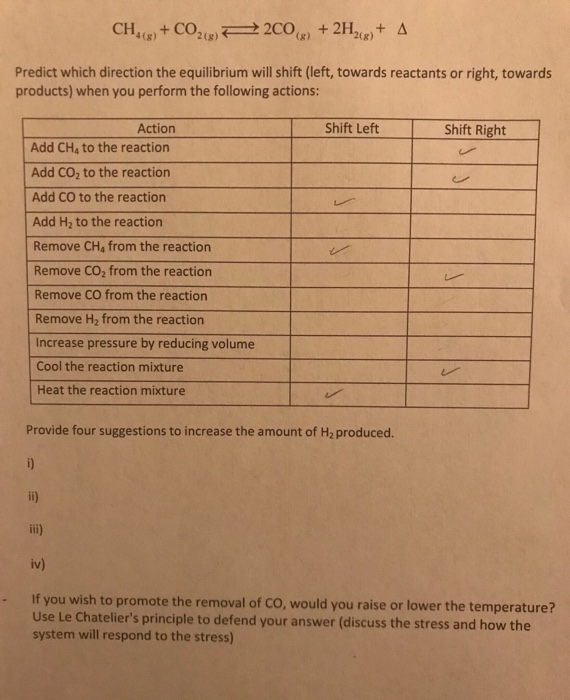+2H2(g)+ CH4(g) +CO2(g)--2CO(g) Δ Predict which direction the equilibrium will shift (left, towards reactants or right, towards products) when you perform the following actions: ft Left Shift Right Action Add CH4s to the reaction Add CO2 to the reaction Add CO to the reaction Add H2 to the reaction Remove CHs from the reaction Remove CO2 from the reaction Remove CO from the reaction Remove H2 from the reaction Increase pressure by reducing volume Cool the reaction mixture Heat the reaction mixture Provide four suggestions to increase the amount of H2 produced. iv) If you wish to promote the removal of CO, would you raise or lower the temperature? Use Le Chatelier's principle to defend your answer (discuss the stress and how the system will respond to the stress) -

This Homework Help Question: "+2H2(g)+ CH4(g) +CO2(g)--2CO(g) Δ Predict which direction the equilibrium will shift (left, towards reactants or right,..." No answers yet.

We need 10 more requests to produce the answer to this homework help question. Share with your friends to get the answer faster!

0 /10 have requested the answer to this homework help question.

Once 10 people have made a request, the answer to this question will be available in 1-2 days.
All students who have requested the answer will be notified once they are available.

#### Earn Coin

Coins can be redeemed for fabulous gifts.

Similar Homework Help Questions
• ### oi Prelab 11, Equilibrium Control of a Reaction me Consider the following equilibrium reaction Predict which...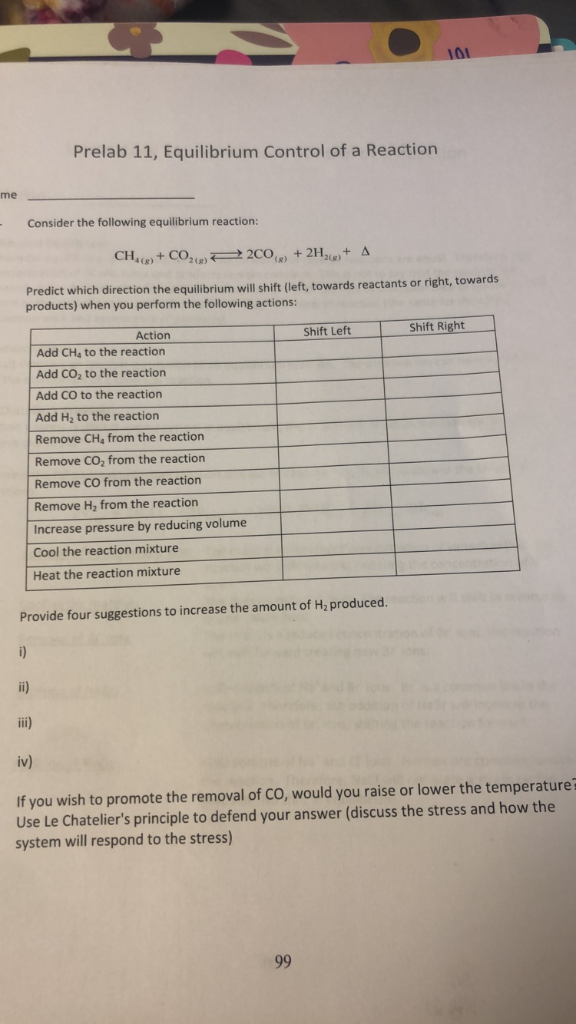oi Prelab 11, Equilibrium Control of a Reaction me Consider the following equilibrium reaction Predict which direction the equilibrium will shift (left, towards reactants or right, towards products) when you perform the following actions: Shift Right Shift Left Action Add CH4 to the reaction Add CO, to the reaction Add CO to the reaction Add H, to the reaction Remove CH4 from the reaction Remove CO2 from the reaction Remove CO from the reaction Remove H2 from the reaction Increase...

• ### Prelab 11, Equilibrium Control of a Reaction Name 1) - Consider the following equilibrium reaction: A+...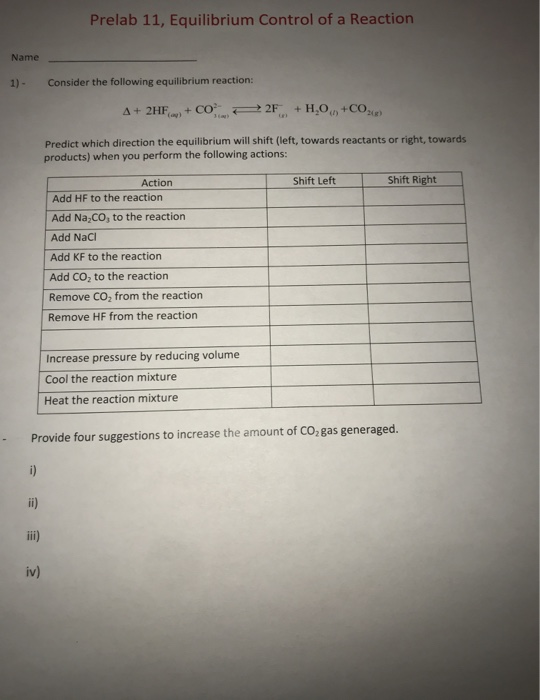Prelab 11, Equilibrium Control of a Reaction Name 1) - Consider the following equilibrium reaction: A+ 2HF + COP 2F+H,O+CO. Predict which direction the equilibrium will shift (left, towards reactants or right, towards products) when you perform the following actions: Shift Left Shift Right Action Add HF to the reaction Add Na,Co, to the reaction Add Naci Add KF to the reaction Add Co, to the reaction Remove COfrom the reaction Remove HF from the reaction Increase pressure by reducing...

• ### Consider this exothermic reaction at equilibrium: C(s) + 2H2(g) - CH2(g). Predict whether the reaction will...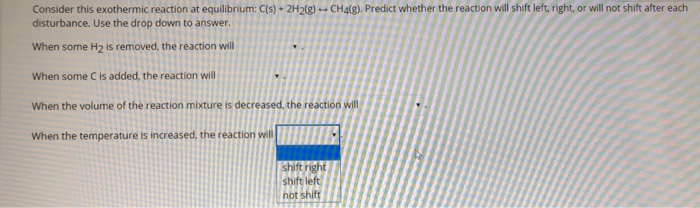Consider this exothermic reaction at equilibrium: C(s) + 2H2(g) - CH2(g). Predict whether the reaction will shift left, right, or will not shift after each disturbance. Use the drop down to answer. When some H2 is removed, the reaction will When some C is added, the reaction will When the volume of the reaction mixture is decreased the reaction will When the temperature is increased, the reaction will shift right shift left not shift

• ### 2. A) Which way will the equilibrium of a reaction shift (towards reactants or products) if...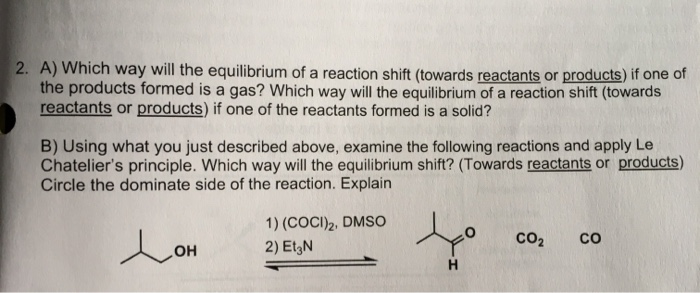2. A) Which way will the equilibrium of a reaction shift (towards reactants or products) if one of the products formed is a gas? Which way will the equilibrium of a reaction shift (towards reactants or products) if one of the reactants formed is a solid? B) Using what you just described above, examine the following reactions and apply Le Chatelier's principle. Which way will the equilibrium shift? (Towards reactants or products) Circle the dominate side of the reaction. Explain...

• ### A mixture of gases is at equilibrium: CH4 (g) + H2O (g) → CO (g) +...

A mixture of gases is at equilibrium: CH4 (g) + H2O (g) → CO (g) + 3 H2 (g) ∆H = 206.103 kJ (a) Does the equilibrium shift to the left or to the right when some H2O (g) is removed from the reaction mixture? (b) Does the equilibrium shift to the left or to the right when some CO (g) is removed from the reaction mixture? (c) In which direction does the equilibrium shift as the temperature is raised?...

• ### Question 7 (5 points) Consider the equilibrium below: CO(g) + H2O(g) = CO2(g) + H2(g) Kc...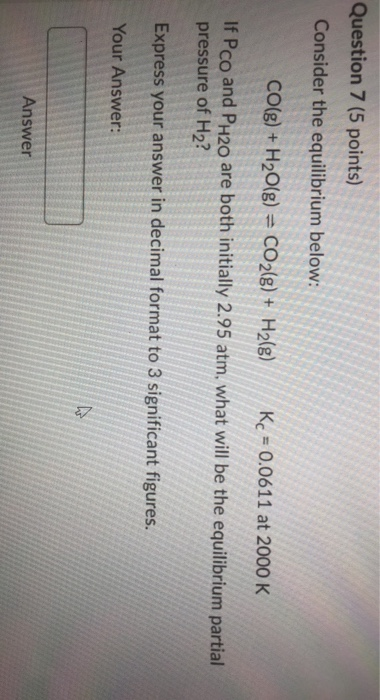Question 7 (5 points) Consider the equilibrium below: CO(g) + H2O(g) = CO2(g) + H2(g) Kc = 0.0611 at 2000 K If Pco and PH20 are both initially 2.95 atm, what will be the equilibrium partial pressure of H2? Express your answer in decimal format to 3 significant figures. Your Answer: N. Answer Coal, which is primarily carbon, can be converted to natural gas (primarily methane, CH4) by the following exothermic reaction: + C(s) 2H2(g) CH4(g) Which of the following...

• ### Consider the following reaction at equilibrium: NiO(s)+CO(g) 2 Ni(s) + CO2(g). Select all of the following...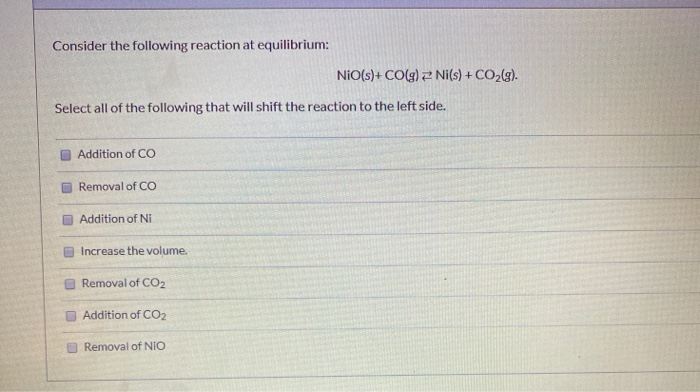Consider the following reaction at equilibrium: NiO(s)+CO(g) 2 Ni(s) + CO2(g). Select all of the following that will shift the reaction to the left side. Addition of CO Removal of CO Addition of Ni Increase the volume. Removal of CO2 Addition of CO2 Removal of NIO Question 1 What is the expression for equilibrium constant for the following reaction? 2 H2S(g) + SO2(g) = 35(s) + 2 H2O(g) Kc= [HQ][S] [SO][H251 Kc= [HO] [H,872 Kc=  [H2 51 (H20 Kc=...

• ### In which direction does the reaction proceed to reach equilibrium? The reaction will proceed from right to left to reach equilibrium. The reaction will proceed from left to right to reach equilibrium.

The first step in the industrial synthesis of hydrogen is the reaction of steam and methane to give synthesis gas, a mixture of carbon monoxide and hydrogen. H2O(g)+CH4(g)⇌CO(g)+3H2(g) Kc = 4.7 at 1400K. A mixture of reactants and products at 1400K contains 0.035 M H2O, 0.050M CH4, 0.15 M CO, and 0.20 M H2. In which direction does the reaction proceed to reach equilibrium? The reaction will proceed from right to left to reach equilibrium. OR The reaction will proceed...

• ### Consider the following equilibrium mixture in a closed system: H2O(g) + CO(g) = H2(g) + CO2(g). Explain what would...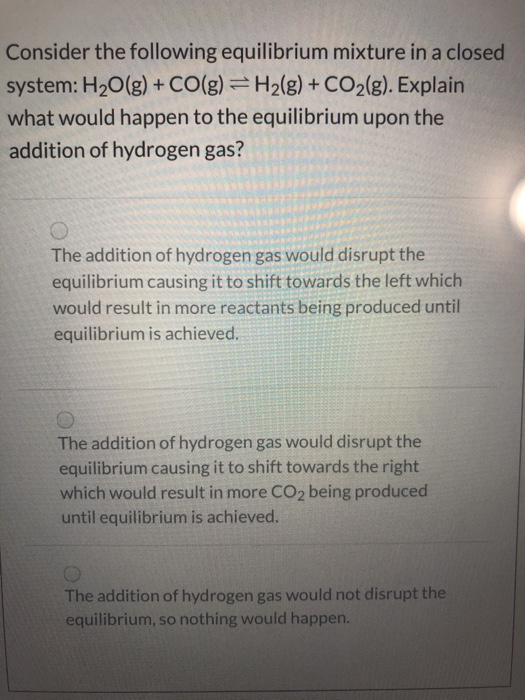Consider the following equilibrium mixture in a closed system: H2O(g) + CO(g) = H2(g) + CO2(g). Explain what would happen to the equilibrium upon the addition of hydrogen gas? The addition of hydrogen gas would disrupt the equilibrium causing it to shift towards the left which would result in more reactants being produced until equilibrium is achieved. The addition of hydrogen gas would disrupt the equilibrium causing it to shift towards the right which would result in more CO2 being...

• ### Consider the following reaction at equilibrium, CaCO3(s) ↔ CaO(s) + CO2(g). Which of the following statements...

Consider the following reaction at equilibrium, CaCO3(s) ↔ CaO(s) + CO2(g). Which of the following statements are true regarding this equilibrium? Select all that are True. a) If CaO(s) is added from the equilibrium mixture the reaction will remain unchanged. b) If CaO(s) is removed from the equilibrium mixture the reaction will shift to the left. c) If CO2(g) is added to the equilibrium mixture the reaction will shift to the right. d) If CO2(g) is added to the equilibrium...